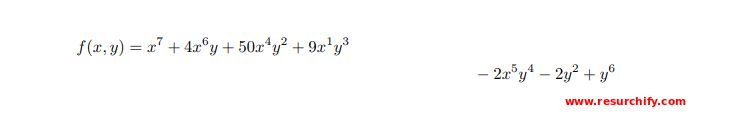Resurchify

Equations alignment using amsmath Package

In LaTeX, amsmath package facilitates many useful features for displaying and representing equations. This package allows you to choose the layout for your document that best suits your requirements. For e.g., you can include multiple equations within the same line and select the layout that best suits your document. You can do this even if the equations are really long, or if you have to include several equations in the same line.

Introduction

The default version of LaTeX may lack some of the functionalities or features. For example, Trimming or Overlapping of equations when equations are very long. To overcome these challenges, you can use the "asmmath" package.

Check the below example to understand:

 1 2 3 4 5 6 \begin{equation} \label{equation1} \begin{split} Area & = \frac{length \times breadth }{2} \\ & = \frac{1}{2} length \times breadth \end{split} \end{equation}Put your equations within an equation environment if you require your equations to get numbered. Otherwise, use equation* (with an asterisk (*) symbol) if you need equations without the line number.

As shown in the example above, utilize the split environment if you would like to split the equations into smaller parts. The split environment will align these smaller parts. Make usage of ampersand (&) character in order to align the equations vertically. Double backslash (\\) provides the functionality of newline character.

Including/Add the amsmath package

It is very easy and straight-forward to include the amsmath package in LaTeX. Use the below command in your document's preamble.
\usepackage{amsmath}

Writing a single equation

Use equation environment in order to print the equation with line number. Otherwise, use equation* environment in order to print the equation without a line number. To reference your equation anywhere in the document, you need to add the \label{...} command as shown below.

 1 2 3 4 5 \begin{equation} \label{circle_area_eq} A=\pi r^{2} \end{equation} The equation \ref{circle_area_eq} represents the area of a circle.Displaying long equations

It is advised to use multline environment in order to print equations that do not fit into a single line. You need to use \\ (Double Backslash) for setting the point where you want to break the equation.

Below example shows how to use the multline environment:

 1 2 3 4 \begin{multline*} f(x,y) = x^7 + 4x^6y + 50x^4y^2 + 9x^1y^3\\ - 2x^5y^4 - 2y^2 + y^6 \end{multline*}Use the equation environment in order to print the equation with the line number. Otherwise, use equation* environment in order to print the equation without a line number. It is important to note that by default, the first part of a broken equation will get left aligned and the second part will get right aligned in the next line.

Equation splitting and alignment

split provides a very similar feature like multline. Just like multline, it is used to break long equations. It aligns the broken part of equations in columns. It is necessary to use the split environment within the equation environment to work properly.

Let's examine an example using split environment:

 1 2 3 4 5 6 \begin{equation} \label{equation1} \begin{split} Area & = \frac{length \times breadth }{2} \\ & = \frac{1}{2} length \times breadth \end{split} \end{equation}Aligning many equations

If you wish to align several equations vertically, then you can use the align environment. Let's check an example using align environment:

 1 2 3 4 \begin{align*} 5x - 1y &= 3 \\ 3x + 7y &= 2 \end{align*}Use the align environment in order to print the equation with the line number. Otherwise, use align* environment in order to print the equation without a line number. Mostly the binary operators (=, > and <) are the ones which are aligned in order to make document beautiful.

As discussed earlier in this tutorial, the ampersand (&) character is used to specify at what point the equations should be aligned. Let's look at below example to understand the alignment of several equations:

 1 2 3 4 5 6 \begin{align*} y&=x & a &=z & b&=a+c\\ 5x&=y & 4z&=\frac{4}{2}z & z&=y\\ 4 + 2x&=1+y & q+2&=4+a & we&=cd\\ x&=y & q &=a & c&=a+b\\ \end{align*}In the above example, we have arranged the equations in three columns. In the above example, it is assumed by the LaTeX that each equation consists of two parts/pieces which are separated by an ampersand (&) character. Also, every equation is isolated using the & from the one previous to it.

Grouping and centering of equations

If you just need to display a set of consecutive equations, centered and with no alignment, use the gather environment. The asterisk trick to set/unset the numbering of equations also works here.

 1 2 3 4 \begin{gather*} 5x^2 - 15y = 8 \\ 3x^2 + 9y = 7 \end{gather*}